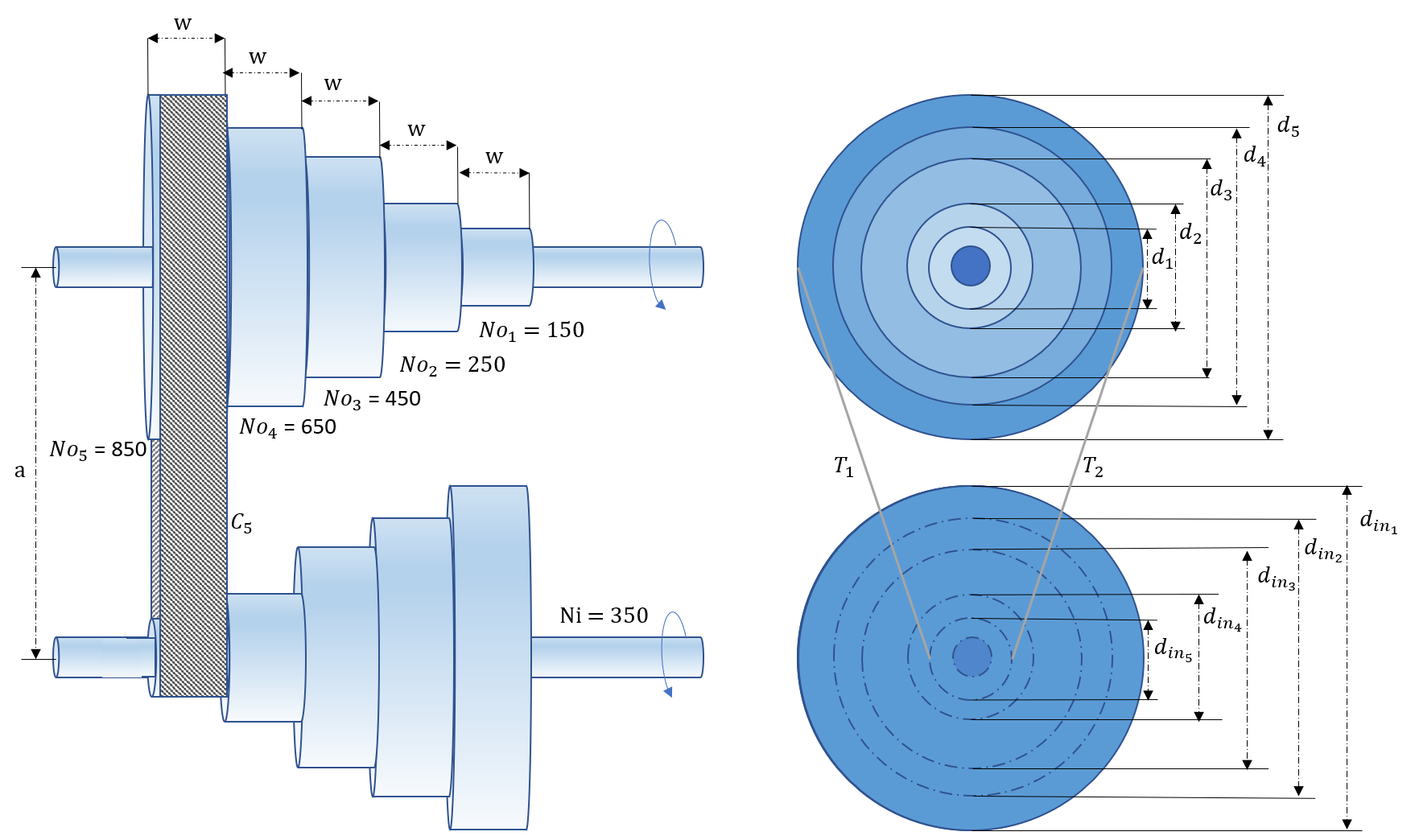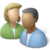### Step Cone Pulley Design Optimization

A step-cone pulley is a type of pulley that consists of two or more conical-shaped pulleys joined together. The conical-shaped pulleys are arranged so that the grooves in each pulley are aligned to mesh with one another, forming a stepped shape. This stepped shape allows for a change in the size of the pulleys without having to change the diameter of the shaft that the pulleys are mounted on. This type of pulley is often used in transmission systems and power take-off drives. A step-cone pulley as shown below in the diagram is used to transmit a power of 0.65 horsepower.The pulley is made up of an aluminum alloy with the density (\rho) of 168.5 \frac{lb}{ft^3}. The belt used is made of Polyamide (A-2) which has a thickness (t) of 0.11 in. and a maximum stress (s) of 75000 \frac{lbf}{ft^2}. The coefficient of friction (\mu) for this model is 0.8. The fixed distance (a) between the centers of the shafts is 1.67 ft. The tension of the tight side of the belt should be at least 2 times that of the the slack side of the belt. The input shaft has a speed of 350 rpm (Ni) and the output shaft should be capable of running at speeds of 950 rpm, 650 rpm, 450 rpm, 250 rpm, and 150 rpm.The design variables are d_i, the diameter of the ith step, and w the width of the belt and each step. The objective of this system is to minimize the weight of the step-cone pulley.

The weight (W) is found by the following equation

$$W = \rho w \frac{\pi}{4} \left(d_1^2 + d_2^2 + d_3^2 + d_4^2 + d_5^2 + d_{in,1}^2 + d_{in,2}^2 + d_{in,3}^2 + d_{in,4}^2 + d_{in,5}^2\right)$$

The diameters of the input pulley (din) are found by using the ratio between the input shaft rpm and the target output rpm.

$$d_{in,i} = d_i \frac{No_i}{N_{in}}$$The belt lengths required for the desired output speeds are determined by the following equation:

$$C_i \simeq \frac{\pi d_i}{2}\left(1 + \frac{No_i}{N_{in}}\right) + \frac{\left(\displaystyle\frac{No_i}{ N_{in}}-1\right)^2d_i^2}{4a},\qquad i = 1,2,3,4,5$$

The angle of the lap of the belt over the ith pulley step is:

$$\theta_i = \pi - 2sin^{-1}\left[\frac{\bigg(\displaystyle\frac{No_i}{ N_{in}}-1\bigg)d_i}{2a}\right]$$

The tension on the tight side of the ith step is given by:

$$T_{1i} = stw$$

The tension of the loose side of the ith step is given by

$$T_{2i} = \frac{T_{1i}}{e^{\mu\theta_i}}$$

The belt length values are subject to the following constraints:

$$C_1 - C_2 = 0$$

$$C_1 - C_3 = 0$$

$$C_1 - C_4 = 0$$

$$C_1 - C_5 = 0$$

The constraint on the power transmitted is given by:

$$\frac{\left(T_{1i} - T_{2i}\right) \pi d_{in,i}(350)}{33000} \ge 0.65$$

The constraint on tension ratio can be given by:

$$e^{ \mu \theta_i} \geq 2$$

Turn in a report with the following sections:

1. Title Page with Summary. The Summary should be short (less than 50 words), and give the main optimization results.
2. Procedure: Give a brief description of your model. You are welcome to refer to the assignment which should be in the Appendix. Also include:
1. A table with the analysis variables, design variables, analysis functions and design functions.
3. Results: Briefly describe the results of optimization (values). Also include:
1. A table showing the optimum values of variables and functions, indicating binding constraints and/or variables at bounds (highlighted)
2. A table giving the various starting points which were tried along with the optimal objective values reached from that point.
4. Discussion of Results: Briefly discuss the optimum and design space around the optimum. Do you feel this is a global optimum? Also include and briefly discuss:
1. A “zoomed out” contour plot showing the design space (both feasible and infeasible) for diameter and thickness, with the feasible region shaded and optimum marked.
2. A “zoomed in” contour plot of the design space (mostly feasible space) for diameter and thickness, with the feasible region shaded and optimum marked.
5. Appendix:
1. Listing of your model with all variables and equations
2. Solver output with details of the convergence to the optimal values

Any output from the software is to be integrated into the report (either physically or electronically pasted) as given in the sections above. Tables and figures should all have explanatory captions. Do not just staple pages of output to your assignment: all raw output is to have notations made on it. For graphs, you are to shade the feasible region and mark the optimum point. For tables of design values, you are to indicate, with arrows and comments, any variables at bounds, any binding constraints, the objective, etc. (You need to show that you understand the meaning of the output you have included.)

Reference

• Singiresu S Rao. Engineering Optimization: Theory and Practice. John Wiley & Sons, 2009.

Acknowledgement

Thanks to Adam Martin for providing the problem statement and the solution.This assignment can be completed in collaboration with others. Additional guidelines on individual, collaborative, and group assignments are provided under the Expectations link.

Solution Helpfrom gekko import GEKKO
m = GEKKO()

# Constants
pi = 3.14159
a = 1.67   # feet
p = 168.5  # density lb/ft^3
u = 0.8
s = 75000  # stress lbf/ft^2
t = 0.0092 # ft

# Variables
w = m.Var(lb=0.01,ub=0.5)
d = [m.Var(lb=1/5) for i in range(5)]
N_o = [950, 650, 450, 250, 150]
N_in = 350

weight = m.Var()

# Intermediates
# Diameter for the ith step of the input pulley
d_in = [m.Intermediate(d[i]*(N_o[i]/N_in)) for i in range(5)]

# Intermediate for objective function
Inter = [m.Intermediate(d[i]**2 + d_in[i]**2) for i in range(5)]

# Belt lengths
C = [m.Intermediate((pi*d[i])/2*(1 + N_o[i]/N_in) \
+ ((((N_o[i]/N_in) - 1)**2)*d[i]**2)/(4*a) \
+ 2*a) for i in range(5)]

# Angles of lap of the belt over the ith pulley step
O = [m.Intermediate(pi - 2*m.asin((((N_o[i]/N_in) \
- 1)*d[i])/(2*a))) for i in range(5)]

# Tensions on the tight side of the ith step (limitation on max tension)
T1 = [m.Intermediate(s*t*w) for i in range(5)]

# Tensions on the slack side of the ith step
T2 = [m.Intermediate(T1[i]/(m.exp(u*O[i]))) for i in range(5)]

# Equations
# Weight, Objective Function
m.Equation(weight == p*w*(pi/4)*(sum(Inter[0:5])))

# Constraints
belt_length = [m.Equation(C - C[i + 1] == 0) for i in range (4)]
tension_ratio = [m.Equation(m.exp(u*O[i]) >= 2) for i in range(5)]
power_transmitted = [m.Equation(((T1[i]-T2[i])*pi*d_in[i]*350)/33000 \
>= 0.65) for i in range(5)]

# Objective and Solve
m.Minimize(weight)

m.options.IMODE = 3
m.options.SOLVER = 1
m.solve()

print('Optimal weight: ' + str(weight))
print('Optimal diameter: ' + str(d))
print('Optimal width: ' + str(w))